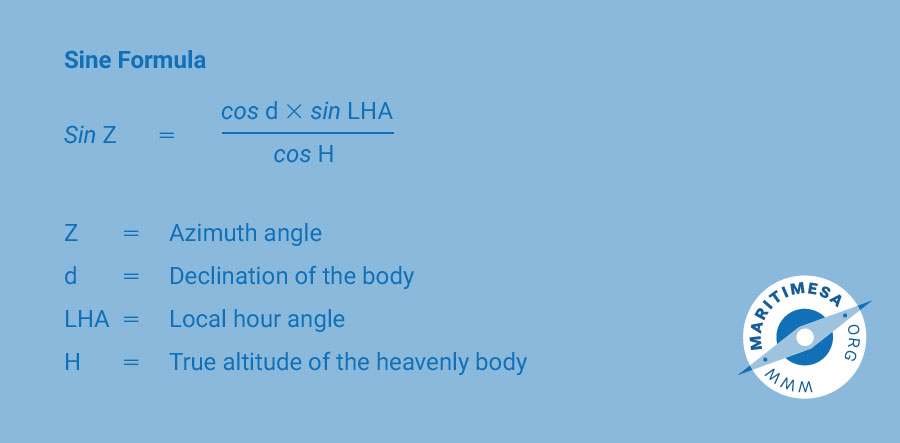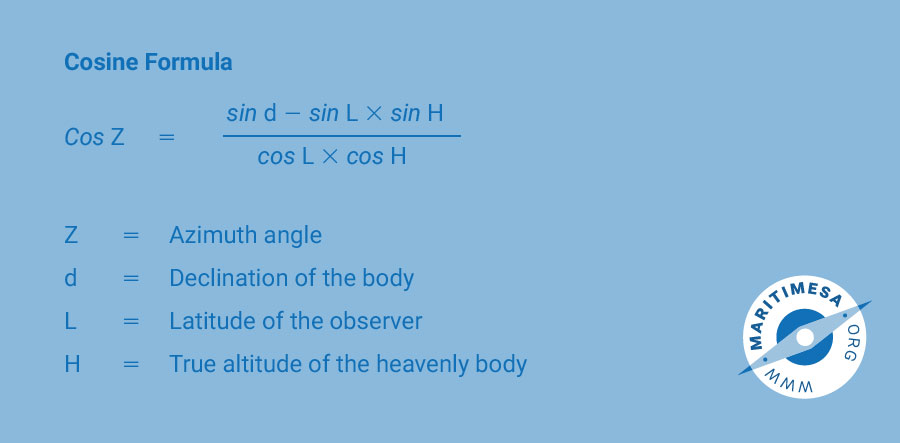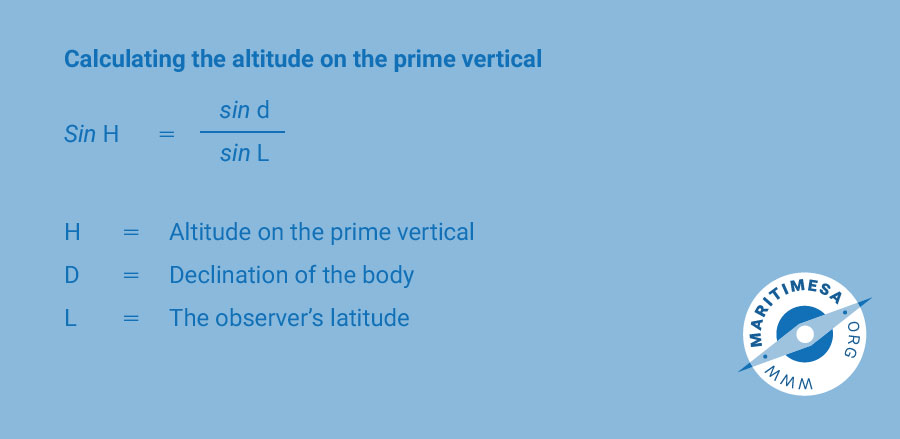# Alternative method of obtaining the sun’s azimuth.

In sub-paragraphs h to k of the procedure, the true bearing is obtained through the use of the A, B, C tables in Nories. There is an alternative method of determining true bearing, namely by calculation. There are two formulas that could be used:Sine formula. In order to convert the azimuth angle to a true bearing, one must determine in which quadrant the body lies (NW, SE, etc). When the LHA is greater than 180°, Z will be prefixed with a minus (-), indicating that it is towards the east. When the LHA is less than 180°, Z will be positive, indicating that it is towards the west. If the body is located near the prime vertical circle (the azimuth is close to 090° or 270°), it may be difficult to decide whether the body is to the north or south. This can be resolved by determining whether the body has crossed the prime vertical circle.

The altitude on the prime vertical can be calculated by using the following formula:If the observed altitude is greater than that on the prime vertical, then the body lies away from the elevated pole. For example, if you are in the southern latitudes, the body lies to the north of the prime vertical and Z will be prefixed “N”.

Cosine formula. This formula always computes Z from true north, provided that south latitude and declination are entered as minus quantities. Unlike the Sine formula, this one does not indicate whether the body lies towards the west or east. The value of the LHA is used to decide this, ie

• In both north and south latitudes, when the LHA is less than 180°, then the azimuth is 360° – Z.
• In both north and south latitudes, when the LHA is greater than 180°, then the azimuth is equal to Z.